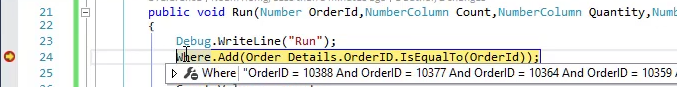﻿ Initialize Values Before the Execution in the Cached Controller

# Initialize Values Before the Execution in the Cached Controller

• Now that we are using `Cached` we are reusing the same instance of the `GetOrderStatistics` class
• We need to adjust the code of the `GetOrderStatistics` class to accommodate the fact that it runs multiple times.
• Add a "Break Point" in the `Run` method, and review the value of `Where` for the several times you run this controller.
• We can see that the where has multiple values### The Where Problem Explained

• In our run method, we do `Where.Add` this means that whenever we run the code, a new condition is added to the Where.
• In first run, the where is: `OrderID = 10388`
• In the Second run, the where is `OrderID = 10388 And OrderID = 10377`
• In the Third run, the where is `OrderID = 10388 And OrderID = 10364 And OrderID = 10377`, this condition doesn't return any row, since no row is both 10388 and 10364 and 10377.
• To Clear the Where, before every execution, we'll use the `Where.Clear` method.
``````public void Run(Number OrderId,NumberColumn Count,NumberColumn Quantity, NumberColumn totalAmount)
{
Where.Clear();
Execute();
Count.Value = _count;
Quantity.Value = _quantity;
totalAmount.Value = _totalAmount;
} ``````
• Now that we've fixed that, we can see that when we are running the code, the values in Items, Total Quantity and Total Amount are always increasing.
• That is because we only initialized them with the value 0, when the class was created and not before every run.
``````Number _count = 0;
Number _quantity = 0;
Number _totalAmount = 0;
protected override void OnLeaveRow()
{
_count++;
_quantity += Order_Details.Quantity;
_totalAmount += Order_Details.Quantity * Order_Details.Quantity.UnitPrice;
} ``````
• So now we need to initialize them before every execution in the `Run` method. Make sure to do that before the call to the `Execute` method in the base class
``````public void Run(Number OrderId,NumberColumn Count,NumberColumn Quantity, NumberColumn totalAmount)
{
Where.Clear();